## 微积分的历史（三），起源之莱布尼兹• 莱布尼兹的微积分思想
• 与近代微积分思想的对比
• 牛顿-莱布尼兹公式之莱布尼兹

1 莱布尼兹的微积分思想

1.1 积分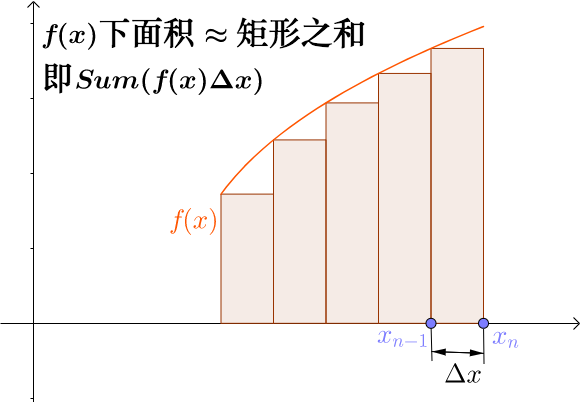Sum就是英文求和的意思，当时没有$\Sigma$这个符号。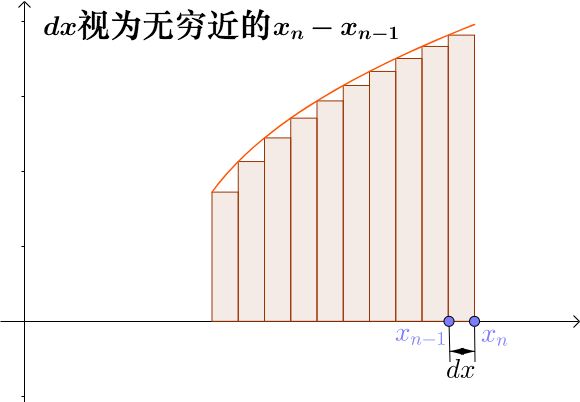$$Sum(f(x)\Delta x)\implies \int _{um} f(x)dx$$

$$\int f(x)dx$$

1.2 切线

Created with GeoGebra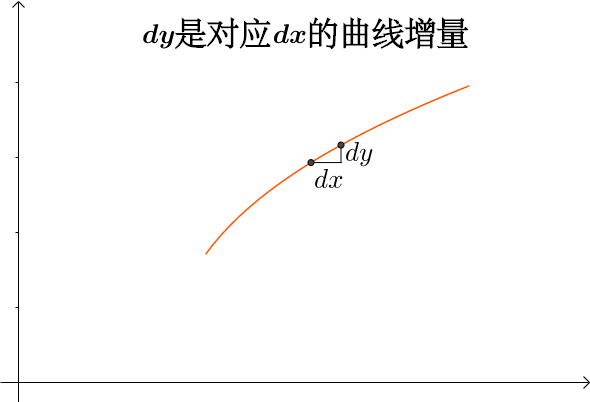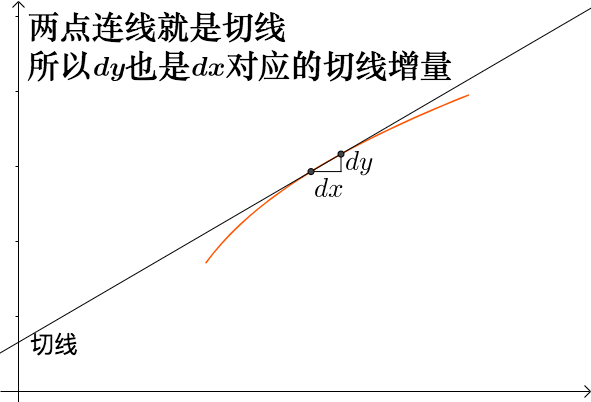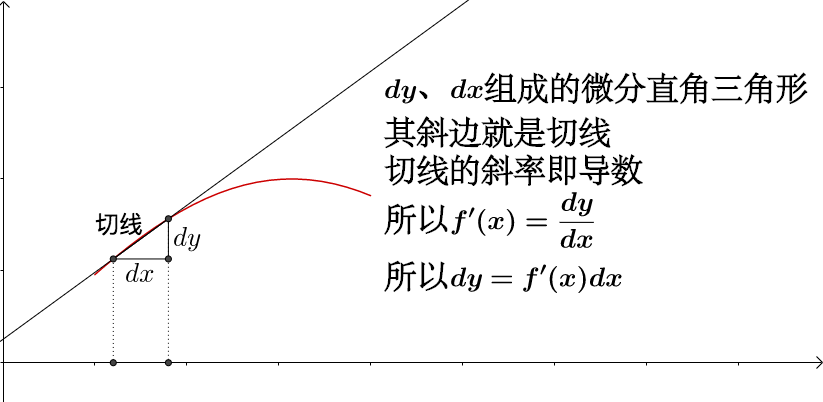1.3 微分

1.4 小结

• 积分：关于无穷小的加法
• $dx$：是无穷小
• $dy$：即是$dx$对应的曲线增量，也是$dx$对应的切线增量
• 切线：通过无穷接近的两个曲线上的点来定义
• 导数：两个无穷小之商，$\frac{dy}{dx}$

2 与近代微积分思想的对比

2.1 无穷小是什么？

2.2 现代微积分观点

2.2.1 切线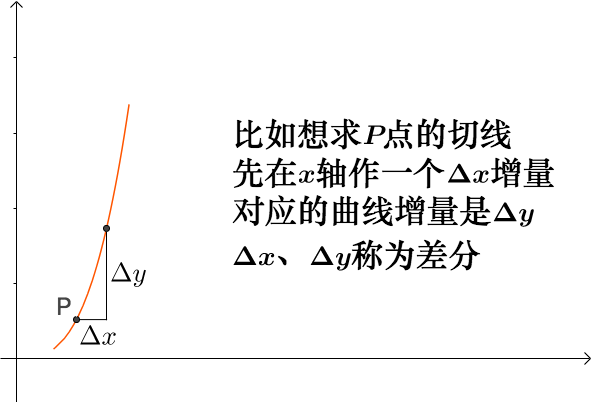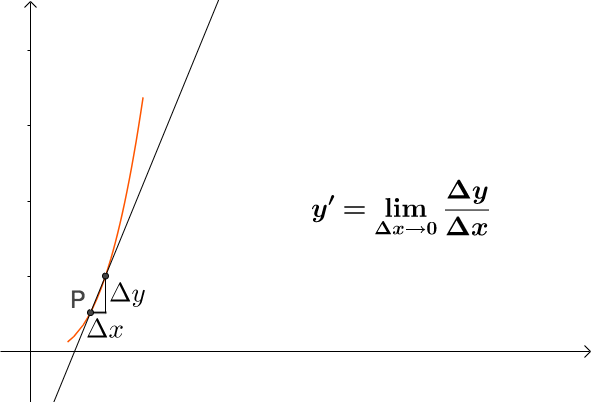2.2.2 微分是什么？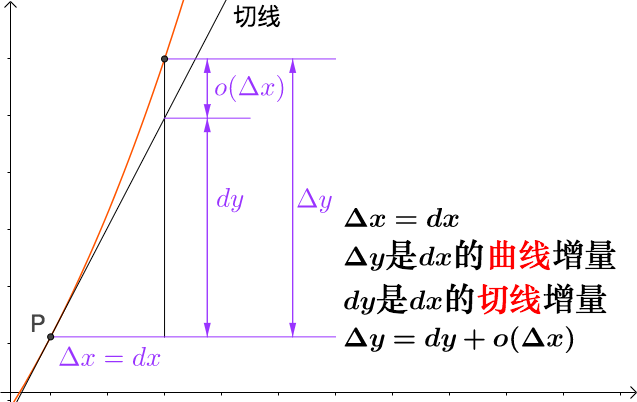2.2.3 以直代曲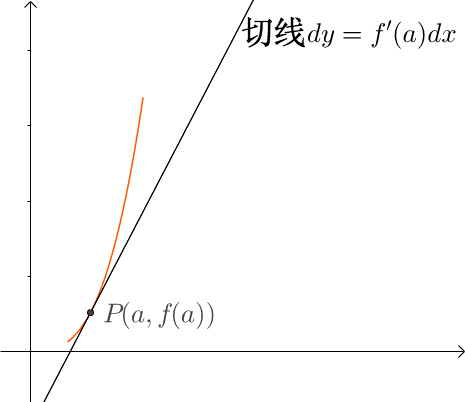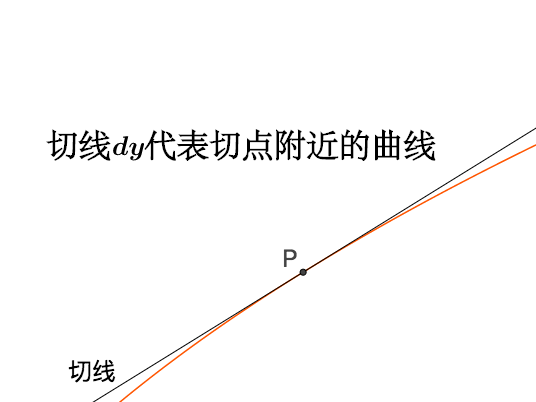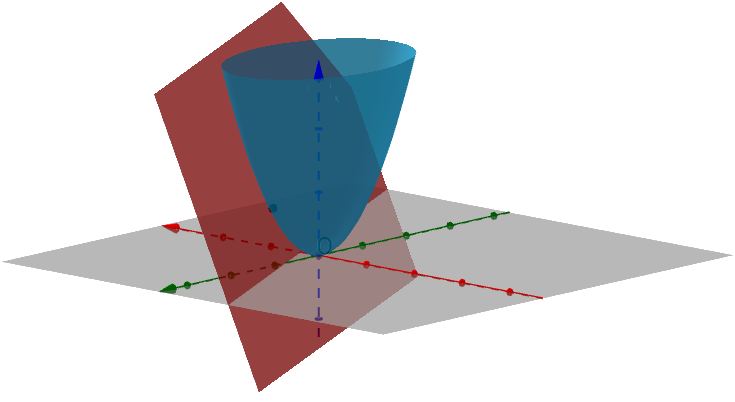2.2.4 积分

2.3 小结

• 积分：黎曼和的极限
• $dx$：$\Delta x$
• $dy$：$dx$对应的切线增量，或者说是切线
• 导数：$\displaystyle y'=\lim _{\Delta x\to 0}\frac{\Delta y}{\Delta x}$
• 切线：根据导数来做出切线

3 牛顿-莱布尼兹公式之莱布尼兹

1677年,莱布尼茨在一篇手稿中正式提出了这一公式。牛顿和莱布尼兹两人共同享有这个公式的命名，以他们在世时水火不容的情况看，不知道这二位是否愿意名字并排在一起。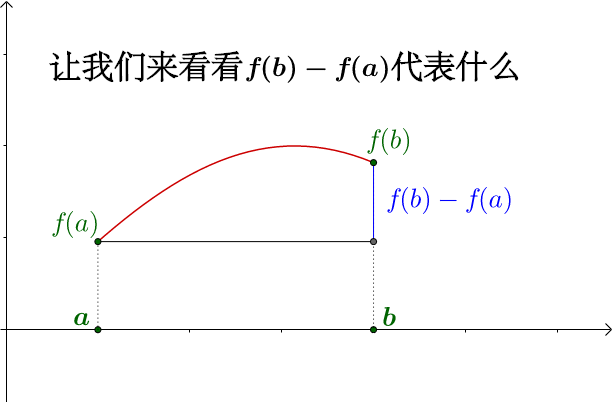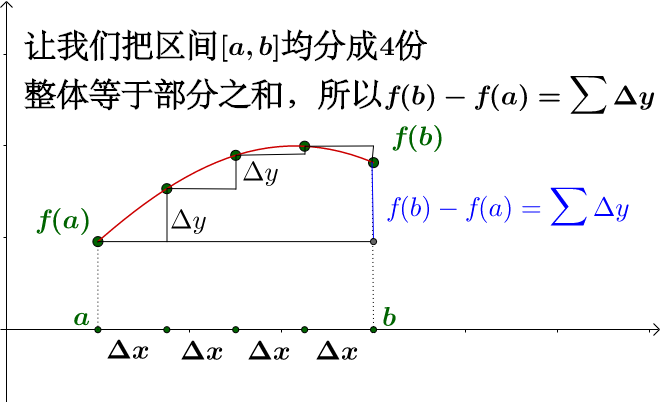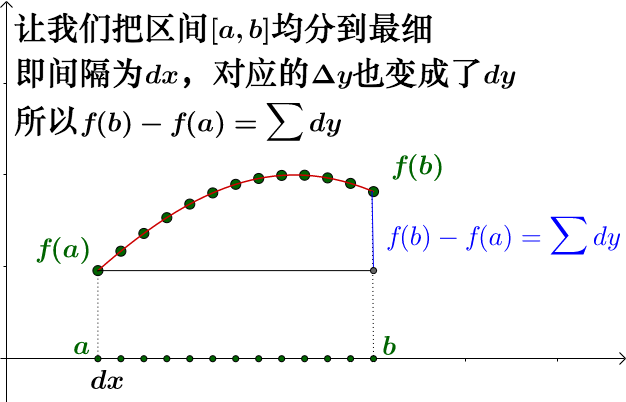$$f(b)-f(a)=\sum f'(x)dx=\int _ a^ b f'(x)dx$$

$f$是$f'$的原函数，从而我们又一次得到了牛顿-莱布尼兹公式。

4 结论

• 微积分的思想脱胎于几何学中的“穷竭法”，但是微积分的思想在两位大师的手中开始独立于几何，这是迈向普遍的第一步
• 微积分开始获得了代数的基础，也就获得了巨大的生命力
• 之前我提到的4类问题，速度、切线、面积、最值，都被微积分统一解决，展现了微积分威力